## Analogy

#### Analogy

Direction: In each of the following questions select the related figure from the given alternatives.

1. Find the question mark ? figure from answer figure.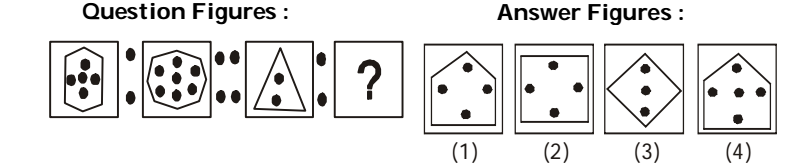1. From first figure to second figure two sides are added to the main design and also two black dots are increased inside the main design.

##### Correct Option: A

From first figure to second figure two sides are added to the main design and also two black dots are increased inside the main design.

1. Find the question mark ? figure from answer figure.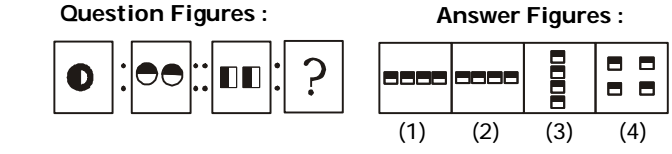1. From first figure to second figure the design rotates through 90° clockwise and becomes double.

##### Correct Option: A

From first figure to second figure the design rotates through 90° clockwise and becomes double.

1. Find the question mark ? figure from answer figure.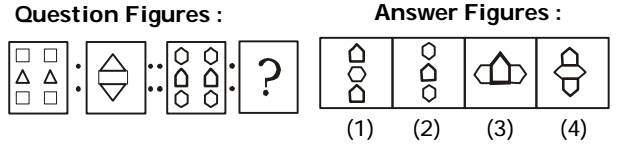1. Out of four rectangles, only one remains in the second figure flanked by the two triangles.

##### Correct Option: D

Out of four rectangles, only one remains in the second figure flanked by the two triangles.

1. Find the question mark ? figure from answer figure.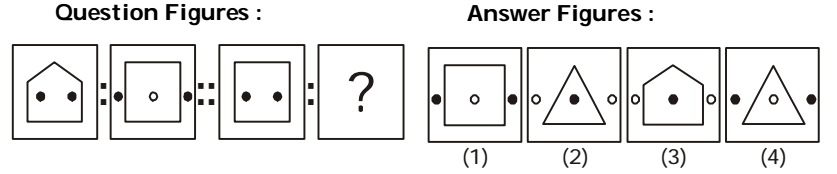1. From first figure to second figure one side of the pentagon is deleted and a square is formed and the two black dots move out of the main design while a small white circle is introduced inside the main design.

##### Correct Option: D

From first figure to second figure one side of the pentagon is deleted and a square is formed and the two black dots move out of the main design while a small white circle is introduced inside the main design.

Direction: In following questions, select the related figure from the given alternatives.

1. Find the question mark ? figure from answer figure.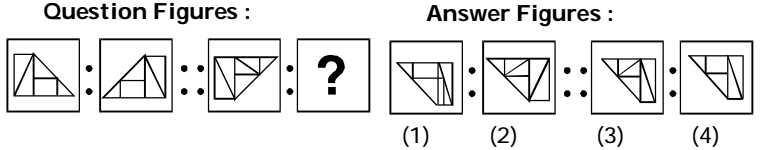1. Second figure in the first pair of Question Figures is mirror image of the first figure.

##### Correct Option: C

Second figure in the first pair of Question Figures is mirror image of the first figure.